Open access to the Encyclopedia has been made possible, in part, with a financial contribution from the Princeton University Library and Philosophy Department. We gratefully acknowledge this support.

# Turing Machines

First published Thu Sep 14, 1995; substantive revision Fri Nov 5, 2004

Turing machines, first described by Alan Turing in (Turing 1937), are simple abstract computational devices intended to help investigate the extent and limitations of what can be computed.

Turing, writing before the invention of the modern digital computer, was interested in the question of what it means to be computable. Intuitively a task is computable if one can specify a sequence of instructions which when followed will result in the completion of the task. Such a set of instructions is called an effective procedure, or algorithm, for the task. This intuition must be made precise by defining the capabilities of the device that is to carry out the instructions. Devices with different capabilities may be able to complete different instruction sets, and therefore may result in different classes of computable tasks (see the entry on computability and complexity).

Turing proposed a class of devices that came to be known as Turing machines. These devices lead to a formal notion of computation that we will call Turing-computability.

The proposition that Turing's notion captures exactly the intuitive idea of effective procedure is called the Church-Turing thesis. This proposition, being a claim about the relationship between a formal concept and intuition, is not provable, though it would be refuted by an intuitively acceptable algorithm for a task that is not Turing-computable. That no such counterexample has been found, together with the fact that Turing-computability is equivalent to independently defined notions of computability based on alternative foundations, such as recursive functions and abacus machines, indicates that there is at least something natural about this notion of computability.

Turing machines are not physical objects but mathematical ones. We require neither soldering irons nor silicon chips to build one. The architecture is simply described, and the actions that may be carried out by the machine are simple and unambiguously specified. Turing recognized that it is not necessary to talk about how the machine carries out its actions, but merely to take as given the twin ideas that the machine can carry out the specified actions, and that those actions may be uniquely described.

## 1. A Definition of Turing Machines

A Turing machine is a kind of state machine. At any time the machine is in any one of a finite number of states. Instructions for a Turing machine consist in specified conditions under which the machine will transition between one state and another.

A Turing machine has an infinite one-dimensional tape divided into cells. Traditionally we think of the tape as being horizontal with the cells arranged in a left-right orientation. The tape has one end, at the left say, and stretches infinitely far to the right. Each cell is able to contain one symbol, either ‘0’ or ‘1’.

The machine has a read-write head, which at any time scanning a single cell on the tape. This read-write head can move left and right along the tape to scan successive cells.

The action of a Turing machine is determined completely by (1) the current state of the machine (2) the symbol in the cell currently being scanned by the head and (3) a table of transition rules, which serve as the “program” for the machine.

Each transition rule is a 4-tuple:

< State0, Symbol, Statenext, Action >

which can be read as saying “if the machine is in state State0 and the current cell contains Symbol then move into state Statenext taking Action”. The actions available to a Turing machine are either to write a symbol on the tape in the current cell (which we will denote with the symbol in question), or to move the head one cell to the left or right, which we will denote by the symbols « and » respectively.

If the machine reaches a situation in which there is not exactly one transition rule specified, i.e., none or more than one, then the machine halts.

In modern terms, the tape serves as the memory of the machine, while the read-write head is the memory bus through which data is accessed (and updated) by the machine. There are two important things to notice about the definition. The first is that the machine's tape is infinite in length, corresponding to an assumption that the memory of the machine is infinite. The second is similar in nature, but not explicit in the definition of the machine, namely that a function will be Turing-computable if there exists a set of instructions that will result in the machine computing the function regardless of the amount of time it takes. One can think of this as assuming the availability of infinite time to complete the computation.

These two assumptions are intended to ensure that the definition of computation that results is not too narrow. This is, it ensures that no computable function will fail to be Turing-computable solely because there is insufficient time or memory to complete the computation. If a function is not Turing-computable it is because Turing machines lack the computational machinery to carry it out, not because of a lack of spatio-temporal resources.

### 1.1. The Definition Formalized

Talk of "tape" and a "read-write head" is intended to aid the intution (and reveals something of the time in which Turing was writing) but plays no important role in the definition of Turing machines. In situations where a formal analysis of Turing machines is required, it is appropriate to spell out the definition of the machinery and program in more mathematical terms. Purely formally a machine might be defined to consist of:
• A finite set of states Q with a distinguished start state,
• A finite set of symbols Σ
A computation state of the machine can be described
• Qstate a member of Q.
• A function σstate from the natural numbers into Σ.
• A natural number hstate.
The transition function for the machine δ is a function from computation states to computation states, such that if δ(S) = T
• σT agrees with σS everywhere except on hS (and perhaps there too.)
• If σS(hS) ≠ σT(hS) then hT = hS otherwise, |hThS| ≤ 1

A formal definition such as this facilitates the analysis of the concept of Turing machine, at the cost of obscuring the underlying intutions about such machines. To see how the formal definition relates to the original one, think of the function σ as representing the tape by assigning an index to each cell of the tape, and then encoding the symbol in that cell as the value of the function σ given the index of the cell.

The read-write head is represented by the number h which is the index of the cell scanned by the head.

The transition function determines the new content of the tape by returning a new function σ but this new function is constrained to differ from the original in at most the cell being scanned. If that cell is modified, then the head must not be moved in the transition, and if it is not modified, then the head is constrained to move at most one cell in either direction.

This definition is very similar to that given in the entry on computability and complexity, with the significant difference that the transition function may write a new symbol as well as move during any transition. This change does not alter the set of Turing-computable functions, and simplifies the formal definition by removing the second condition on the transition function in our definition. Both formal definitions permit the alphabet of symbols on the tape to be any finite set, while the original definition insisted on Σ={0,1} this change also does not impact the definition of the set of Turing-computable functions.

## 2. Describing Turing Machines

Every Turing machine has the same machinery. What makes one Turing machine perform one task and another a different task is the table of transition rules that make up the machine's program, and a specified initial state for the machine. We will assume throughout that a machine starts in the lowest numbered of its states.

We can describe a Turing machine, therefore, by specifying only the 4-tuples that make up its program. Here are the tuples describing a simple machine.

 < s0, 0, s1, 1 > < s1, 1, s1, « > < s1, 0, s2, » >

When we are interested in examining the behaviour of a Turing machine, it is common and perhaps more perspicuous to represent the machine using a state diagram. Here is the machine represented in this format.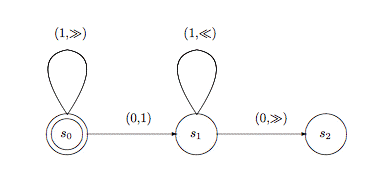Figure 1: A State Diagram

In this figure, states are represented by the circles, with the unique double circle being the initial state. A transition is represented as an arrow originating from one circle and landing at another (possibly the same) circle. The arrows are labeled by a pair consisting first of the symbol that must be being scanned for the arrow to be followed, and second the action that is to be taken as the transition is made. The action will either be the symbol to be written, or « or » indicating a move to the left or right.

In what follows we will describe Turing machines in the state machine format.

### 2.1 Examples

In order to speak about a Turing machine that does something useful, we will have to provide an interpretation of the symbols recorded on the tape. For example, if we want to design a machine which will perform some mathematical function, addition say, then we will need to describe how to interpret the ones and zeros appearing on the tape as numbers.

In the examples that follow we will represent the number n as a block of n+1 copies of the symbol ‘1’ on the tape. Thus we will represent the number 0 as a single ‘1’ and the number 3 as a block of four ‘1’s.

We will also have to make some assumptions about the configuration of the tape when the machine is started, and when it finishes, in order to interpret the computation. We will assume that if the function to be computed requires n arguments, then the Turing machine will start with its head scanning the leftmost ‘1’ of a sequence of n blocks of ‘1’s. the blocks of ‘1's representing the arguments must be separated by a single occurrence of the symbol ‘0’. For example, to compute the sum 3+4, a Turing machine will start in the following configuration, where the ellipses indicate that the tape has only zeros on the cells that we can't see, and the upward arrow indicates the cell that is currently scanned.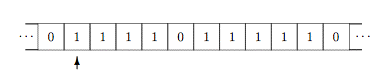A machine must finish in standard configuration too. There must be a single block of ‘1’s on the tape, and the machine must be scanning the leftmost such ‘1’. If the machine correctly computes the function then this block must represent the correct answer. So an addition machine started in the configuration above must finish on a tape that looks like this: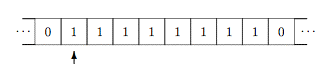Adopting this convention for the terminating configuration of a Turing machine means that we can compose machines by identifying the final state of one machine with the initial state of the next.

Under these conventions, the state diagram in Figure 1 describes a machine which computes the successor (add-one) function. That is when started in standard configuration on a tape representing the number n it will halt in standard configuration representing the number n+1. It does this by using state s0 to scan to the first ‘0’ to the right of the (single) block of ‘1’s. It then replaces that ‘0’ by a ‘1’, and scans left in state s1 until a ‘0’ is found (this is the first zero to the left of the block of ‘1’s.) It then moves back to scan the first ‘1’ and halts in state s2.

You can see a movie of the execution of this machine here.

For another example, consider the machine in Figure 2 which computes the addition function. That is, when started on a standard tape representing the numbers n and m, the machine halts on a tape representing n+m.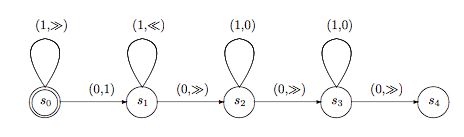Figure 2: A Machine for Computing n+m
Notice that this machine is like the add one machine in that states s0 through s 2 cause the machine to write a ‘1’ to the right of the first block of ‘1’s, and returns the head to the leftmost ‘1’. In standard configuration for addition, this joins the two blocks of ‘1’s into a single block, containing (n+1)+1+(m+1) copies of the symbol ‘1’, so that on entering state s2 the tape represents the number n+m+2. In order to correct this, we need to remove two copies of the symbol ‘1’, which is achieved by states s 2 and s3, each of which replaces a ‘1’ by ‘0’ and then moves to the right.

Since the tape initially contains at least two ‘1’s and we write one more, the deletion of two ‘1’s will leave at least one on the tape at the end of the computation, and we will be scanning the leftmost of them.

### 2.2 Instantaneous Descriptions of a Computation

The complete state of a Turing machine at any point during a computation may be described by the name of the state that the machine is in, the symbols on the tape, and the cell that is currently being scanned. A description of these three data is called an instantaneous description of the computation. We will represent such a description using a figure like that in Figure 3, in which the arrow represents the currently scanned cell and the name of the current state is written below the arrow.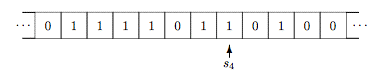Figure 3: The Instantaneous Description of a Turing Machine Computation

## 3. Varieties of Turing Machines

We have presented here one of the most common formulations of Turing's basic idea. There are a number of variations to the formulation that turn out to be equivalent to this one, and different authors present Turing machines using any of these. Since they are all provably equivalent to one another we can consider any of the formulations as being the definition of Turing machine as we find convenient.

Formulation F1 and formulation F2 are equivalent if for every machine described in formulation F1 there is machine a described in F2 which has the same input-output behavior, and vice versa, i.e. when started on the same tape at the same cell, will terminate with the same tape on the same cell.

Two-way infinite tapes

In our original formulation we specified that the tape had an end, at the left say, and stretched infinitely far to the right. Relaxing this stipulation to allow the tape to stretch infinitely far to right and left results in a new formulation of Turing machines equivalent to the original. That is for any Turing machine using a two way tape there is a Turing machine with a one-way infinite tape with the same input-output behavior, and vice versa.

Two-dimensional tapes

Instead of a one-dimensional infinite tape, we could consider a two-dimensional “tape”, which stretches infinitely far up and down as well as left and right. We would add to the formulation that a machine transition can cause the read-write head to move up or down one cell in addition to being able to move left and right. Again this formulation is equivalent to the original.

Modifying the definition of a Turing machine so that the read-write head may move an arbitrary number of cells at any given transition does not alter the notion of Turing-computability.

Modifying the definition of a Turing machine so that the machine has several read-write heads does not alter the notion of Turing-computability.

Arbitrary finite alphabet

In our original formulation we allowed the use of only two symbols on the tape. In fact we do not increase the power of Turing machines by allowing the use of any finite alphabet of symbols.

5-tuple formulation

A common way to describe Turing machines is to allow the machine to both write and move its head in the same transition. This formulation requires the 4-tuples of the original formulation to be replaced by 5-tuples

<State0, Symbol, Statenew, Symbolnew, Move >

where Symbolnew is the symbol written, and Move is one of « and ».

Again, this additional freedom does not result in a new definition of Turing-computable. For every one of the new machines there is one of the old machines with the same properties.

Non-deterministic Turing machines

An apparently more radical reformulation of the notion of Turing machine allows the machine to explore alternatives computations in parallel. In the original formulation we said that if the machine specified multiple transitions for a given state/symbol pair, and the machine was in such a state then it would halt. In this reformulation, all transitions are taken, and all the resulting computations are continued in parallel. One way to visualize this is that the machine spawns an exact copy of itself and the tape for each alternative available transition, and each machine continues the computation. If any of the machines terminates successfully, then the entire computation terminates and inherits that machine's resulting tape. Notice the word successfully in the preceding sentence. In this formulation, some states are designated as accepting states and when the machine terminates in one of these states, then the computation is successful, otherwise the computation is unsuccessful and any other machines continue in their search for a successful outcome.

The addition of non-determinism to Turing machines does not alter the definition of Turing-computable.

Turing's original formulation of Turing Machines used the 5-tuple representation of machines. Post introduced the 4-tuple representation, and the use of a two-way infinite tape.

A more complex machine

In addition to performing numerical functions using unary representation for numbers, we can perform tasks such as copying blocks of symbols, erasing blocks of symbols and so on. Here is an example of a Turing machine which when started in standard configuration on a tape containing a single block of ‘1’s, halts on a tape containing two copies of that block of ‘1’s, with the blocks separated by a single ‘0’. It uses an alphabet consisting of the symbols ‘0’, ‘1’ and ‘A’.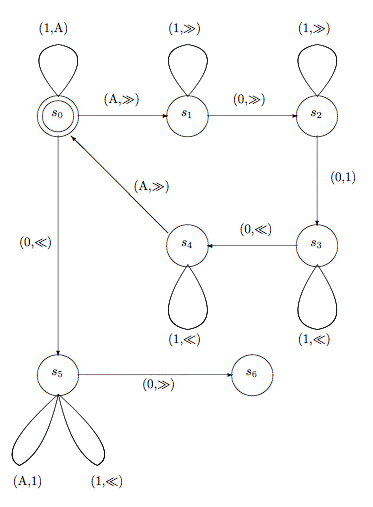Figure 4: A Machine for Copying a Block of 1s
The action of this machine is to repeatedly change one of the original ones into an A, and then write a new ‘1’ to the right of all remaining ‘1’ on the tape, after leaving a zero between the original block and the copy. When we run out of the original ‘1’s, we turn the As back into ‘1’s.

The initial state, s0, is used to change a ‘1’ into an ‘A’, and move to the right and into state s1. In state s1 we skip the remainder of the block of ‘1’s until we find a ‘0’ (the block separator) and in s2 we skip any ‘1’s to the right of that ‘0’ (this is the copy of the block of ‘1’s that we are making.). When we reach the end of that block, we find a ‘0’, which we turn into a ‘1’ and head back to the left, and into state s3. States s3 and s4 skip leftward over the ‘1’s and separating ‘0’ on the tape until an ‘A’ is found. When this occurs, we go back into state s0, and move rightward.

At this point, we are either scanning the next ‘1’ of the original block, or the original block has all been turned into ‘A's, and we are scanning the separator ‘0’. In the former case, we make another trip through states s1s4, but in the latter, we move into state s5, moving leftward. In this state we will repeatedly find ‘A's, which we replace with ‘1’s, and move to the left. If we find a ‘0’, then all of the ‘A's have been turned back into ‘1’s. We will be scanning the ‘0’ to the left of the original cell, and so we move right, and into the final state s6.

This copying machine could be used in conjunction with the addition machine of Figure 2 to construct a doubling machine, i.e. a machine which, when started on a tape representing the number n halts on a tape representing 2n. We could do this by first using the copying machine to produce a tape with two copies of n on the tape, and then using the addition machine to compute n+n (=2n). We would do this by identifying the copying machine's halt state (s6) with the adding machine's initial state (s0).

The construction just suggested relies on the fact that the copying machine terminates in standard position, which is required for the adding machine to correctly compute its result. By designing Turing machines which start and end in standard configuration, we can ensure that they may be composed in this manner. In the example, the copying machine has a unique terminating state, but this is not necessary. We might build a Turing machine which indicates the result of its computation by terminating on one of many states, and we can the combine that machine with more that one machine, with the identity of the machine which follow dependent on the switching machine. This would enable us to create a machine which adds one to the input if that input is even, and doubles it if odd, for example (should we want to for some reason).

## 4. What Can Be Computed

Turing machines are very powerful. For a very large number of computational problems, it is possible to build a Turing machine that will be able to perform that computation. We have seen that it is possible to design Turing machines for arithmetic on the natural numbers, for example.

Computable Numbers

Turing's original paper concerned computable numbers. A number is Turing-computable if there exists a Turing machine which starting from a blank tape computes an arbitrarily precise approximation to that number. All of the algebraic numbers (roots of polynomials with algebraic coefficients) and many transcendental mathematical constants, such as e and π are Turing-computable.

Computable Functions

As we have seen, Turing machines can do more than write down numbers. Among other things they can compute numeric functions, such as the machine for addition (presented in Figure 2) multiplication, proper subtraction, exponentiation, factorial and so on.

By adopting a convention for representing TRUE and FALSE, perhaps that TRUE is represented as a sequence of two ‘1’s and FALSE as one ‘1’, we can compute the characteristic functions of computable predicates, and we may combine the results of such functions using the boolean functions: AND, NOT, OR, IF-THEN-ELSE.

In fact the Turing-computable functions are just the recursive functions, described below.

Universal Turing Machines

The most striking positive result concerning the capabilities of Turing machines is the existence of Universal Turing Machines (UTM). When started on a tape containing the encoding of another Turing machine, call it T, followed by the input to T, a UTM produces the same result as T would when started on that input. Essentially a UTM can simulate the behavior of any Turing machine (including itself.)

One way to think of a UTM is as a programmable computer. When a UTM is given a program (a description of another machine), it makes itself behave as if it were that machine while processing the input.

Note again, our identification of input-output equivalence with “behaving identically”. A machine T working on input t is likely to execute far fewer transitions that a UTM simulating T working on t, but for our purposes this fact is irrelevant.

In order to design such a machine, it is first necessary to define a way of representing a Turing machine on the tape for the UTM to process. To do this we will recall that Turing machines are formally represented as a collection of 4-tuples. We will first design an encoding for individual tuples, and then for sequences of tuples.

Encoding Turing Machines

Each 4-tuple in the machine specification will be encoded as a sequence of four blocks of ‘1’s, separated by a single ‘0’

1. The first block of ones will encode the current state number, using the unary number convention above (n+1 ones represents the number n).
2. The second block of ones will encode the current symbol, using one ‘1’ to represent the symbol zero, and two to represent the symbol ‘1’ (again because we can't use zero ones to represent ‘0’.)
3. The third element of the tuple will represent the new state number in unary number notation.
4. The fourth element represents the action, and there are four possibilities: symbols will be encoded as above, with a block of three ‘1’s representing a move to the left («) and a block of four ‘1’s representing a move to the right (»).

Using this convention the tuple <0, ‘1’, 0, »> would be represented as in Figure 4.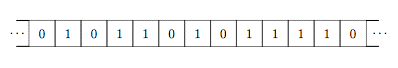Figure 4: The Encoding of the Tuple <0, ‘1’, 0, »>

To encode a complete machine, we need to simply write down the tuples on the tape, in any order, but separated from one another by two blank cells. The add-one machine of Figure 1, would be represented by the somewhat intimidating string shown in Figure 5.

… 010110101111 00 101011011 00 110110110111 00 11010111011110 …

Figure 5: The Encoding of the Machine in Figure 1

If we interpret encodings such as that in Figure 5 as a binary number (ignoring trailing zeros), we see that each Turing machine has a serial number.

## 5. What Cannot Be Computed

A crucial observation about Turing machine is that there are only countably many machines (a set is countable if it is finite, or may be placed in one-to-one correspondence with the integers.) It follows immediately that there are uncountably many real numbers that are not computable, since the reals are not countable. There are simply not enough Turing machines to compute all of those numbers.

Like the real numbers, the number of functions on the natural numbers is not countable. It follows therefore that there are uncountably many such functions that are not computable by any Turing machine.

Here we give two examples of uncomputable functions.

### 5.1 The Busy Beaver

Define the productivity of a Turing machine as the number of ones on the tape when it halts in standard configuration, if it does, and 0 if it does not. The machine is assumed to start in its lowest number state and with an initially blank tape. Now define p(n) to be the productivity of the most productive n-state machine. There is no Turing machine which will compute the function p, i.e., which when started in standard configuration on a tape with n ‘1’s will halt in standard configuration on a tape with p(n) ‘1’s. This example is due to Tibor Radó (Radó 1962).

We can prove the uncomputability of the busy beaver function by deriving a contradiction from the assumption that such a machine exists. The crucial step in the proof, is to note that if the Busy Beaver machine were to exist, the productivity of the most productive n+2k state machine is at least p(p(n)), where k is the number of states of the (alleged) Busy Beaver machine. This is demonstrated by building a machine which initially writes n ‘1’s on the tape (which can be done in n states — exercise for the reader), and then connecting the halt state of that machine to the start state of the Busy Beaver machine (resulting in a computation of p(n)), and then connecting the halt state of that machine with another copy of the Busy Beaver machine, resulting a computation of p(p(n)). Thus (if the Busy Beaver machine exists)

p(n+2k) ≥ p(p(n)) for any n.
It is easy to show that the productivity of Turing machines increases as states are added, i.e.
if i < j, then p(i) < p(j)
(another exercise). Consequently (if the Busy Beaver machine exists)
n+2k ≥ p(n) for any n.
Since this is true for any n, it is true for n+11, yielding:
n+11+2k ≥ p(n+11) for any n.
But it is easy to show that p(n+11) ≥ 2n (another exercise, but show that there is an eleven state machine for doubling the number of ‘1’ on the tape, and compose such a machine with the n-state machine for writing n ‘1’s). Combining this fact with the previous inequality we have:
n+11+2k ≥ p(n+11) ≥ 2n for any n.
from which by subtracting n from both sides we have 11+2kn for any n if the Busy Beaver exists, which is a contradiction.

Even though the productivity function is uncomputable, there is considerable interest in the search for Busy Beaver Turing machines (most productive machines with a given number of states). Some candidates can be found by following links in the Other Internet resources section of this article.

### 5.2 The Halting Problem

It would be very useful to be able to examine the description of a Turing machine and determine whether it halts on a given input. this problem is called the Halting problem and is, regrettably, uncomputable. That is, no Turing machine exists which computes the function h(t,n) which is defined to be TRUE if machine t halts on input n and FALSE otherwise.

To see the uncomputability of the halting function, imagine that such a machine H exists, and consider a new machine built by composing the copying machine of Figure 4 with H by joining the halt state of the copier to the start state of H. Such a machine, when started on a tape with n ‘1’s determines whether the machine whose code is n halts when given input n, i.e. it computes M(n) = h(n,n).

Now lets add another little machine to the halt state of H. This machine goes into an infinite sequence of transitions if the tape contains TRUE when it starts, and halts if the tape contains FALSE (its an exercise for the reader to construct this machine, assume that TRUE is represented by ‘11’, and FALSE by ‘1’.)

This composed machine, call it M, halts if the machine with the input code n does not halt on an initial tape containing n (because if machine n does not halt on n, the halting machine will leave TRUE on the tape, and M will then go into its infinite sequence.) and vice versa.

To see that this is impossible, consider the code for M itself. What happens when M is started on a tape containing Ms code? Assume that M halts on M, then by the definition of the machine M it does not halt. But equally, if it does not halt on M the definition of M says that it should halt.

This is a contradiction, and the Halting machine cannot exist. The fact that the halting problem is not Turing-computable was first proved by Turing in (Turing 1937).

## 6. Alternative Formulations of Computability

### 6.1 Recursive Functions

Recursive function theory is the study of the functions that can be defined using recursive techniques (see the entry on recursive functions). Briefly, the primitive recursive functions are those that can be formed from the basic functions:

 the zero function: z(x) = 0, for all x the successor function: s(x) = x+1, for all x the ith projection over j arguments: pi,j(x0,…xj) = xi, for all xi,i,j

by using the operations of composition and primitive recursion.

 Composition: f(x1,…,xn) = g(h1(x1,…,xn),…, hm(x1,…,xn)) for all g,h1,…,hm Primitive Recursion: f(x,0) = g(x) for any g f(x,s(y)) = h(x,y,f(x,y)) for any h
The recursive functions are formed by the addition of the minimization operator, which takes a function f and returns h defined as follows:
 Minimization: h(x1, …,xn) = y, if f(x1, …,xn,y)=0 and ∀ t < y. f(x1, …,xn,t) is defined and positive. = undefined otherwise.

It is known that the Turing computable functions are exactly the recursive functions.

### 6.2 Abacus Machines

Abacus machines abstract the more familiar architecture of the modern digital computer (the von Neumann architecture.) In its simplest form a computer with such an architecture has a number of addressable registers each of which can hold a single datum, and a processor which can read and write to these registers.

The machine can perform two basic operations, namely: add one to the content of a named register (which we will symbolize as n+, where n is the name of the register) and (attempt to) subtract one from a named register, with two possible outcomes: a success branch if the register was initially non-zero, and a failure branch if the register was initially zero (we will symbolize the operation as n-).

These are called abacus computers by Lambek (Lambek 1961), and are known to be equivalent to Turing machines.

The modern digital computer is subject to finiteness constraints that we have abstracted away in the definition of abacus machines, just as we did in the case of Turing machines. Physical computers are limited in the number of memory locations that they have, and in the storage capacity of each of those locations, while abacus machines are not subject to those constraints. Thus some abacus-computable functions will not be computable by any physical machine. (We won't consider whether Turing machines and modern digital computers remain equivalent when both are given external inputs, since that would require us to change the definition of a Turing machine.)

## 7. Restricted Turing Machines

One way to modify the definition of Turing machines is by removing their ability to write to the tape. The resulting machines are called finite state machines. They are provably less powerful than Turing machines, since they cannot use the tape to remember the state of the computation. For example, finite state machines cannot determine whether an input string consists of some As followed by the same number of Bs. The reason is that the machine cannot remember how many As it has seen so far, except by being in a state that represents this fact, and determining whether the number of As and Bs match in all cases would require the machine to have infinitely many states (one to remember that it has seen one A, one to remember that it has seen 2, and so on.)

## Bibliography

• Barwise, J. and Etchemendy, J., 1993, Turing's World, Stanford: CSLI Publications.
• Boolos, G.S. and Jeffrey, R.C., 1974, Computability and Logic, Cambridge: Cambridge University Press.
• Davis, M., 1958, Computability and Unsolvability, McGraw-Hill; reprinted Dover 1982.
• Herken, R., (ed.), 1988, The Universal Turing Machine: A Half-Century Survey, New York: Oxford University Press.
• Hodges, A., 1983, Alan Turing, The Enigma, New York: Simon and Schuster.
• Kleene, S.C., 1936, "General recursive Functions of Natural Numbers". Mathematische Annalen, 112.
• Lambek, J., 1961, "How to program an infinite abacus", Canadian Mathematical Bulletin, 4: 279–293.
• Lewis, H.R. and Papadimitriou, C.H., 1981, Elements of the Theory of Computation, Prentice-Hall.
• Lin, S. and Radó, T., 1965, "Computer Studies of Turing Machine Problems," Journal of the Association for Computing Machinery, 12, 196–212.
• Post, E., 1947, "Recursive Unsolvability of a Problem of Thue," The Journal of Symbolic Logic, 12.
• Radó, T., 1962, "On Non-computable functions", Bell System Technical Journal, May.
• Turing, A.M., 1936-7, "On Computable Numbers, With an Application to the Entscheidungsproblem", Proceedings of the London Mathematical Society, (2) 42, pp 230-265; correction ibid. 43, pp 544-546 (1937). [Available Online].
• Turing, A.M., 1937, "Computability and λ-Definability", The Journal of Symbolic Logic, 2.

## Other Internet Resources

### Online Turing Machine Simulators

There are many Turing machine simulators available. Here are three that use different technologies to implement simulators using your browser.
Here are some applications that you can run on the desktop (no endorsement of these programs is implied).
• Turing's World: a courseware package available from CSLI Publications (Macintosh only)
• Visual Turing: freeware simulator for Widnows 95/98/NT/2000
During the second world war, Alan Turing was involved in code breaking activites at Station X in Bletchley Park in the UK.

## Related Entries

Church, Alonzo | Church-Turing Thesis | computability and complexity | function: recursive | Turing, Alan